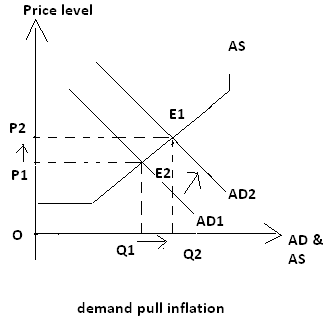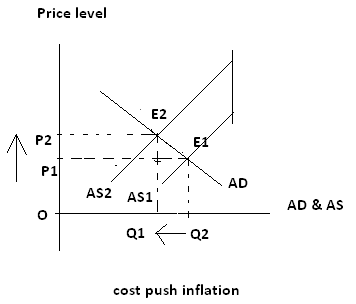# Inflation

It is defined as persistent rise in price level. During inflation the goods and services become more and more expensive in average, as value of money decreases with increases in price level. The inflation can be defined as the persistent fall in value of money too. Inflation is long term rise in price level. The temporal rise in price level is not said to be inflation. Rise in price level should be for more years to be inflation. The inflation is caused by more aggregate demand than aggregate supply. During inflation, the goods and services demanded in the country are more than goods and services supplied. If aggregate demand is more than aggregate supply due to any reason, the price level rises. The process continuously takes place till the aggregate demand remains more than aggregate supply.

There are mainly two types of inflation they are

1. Demand- pull inflation:

The persistent rise in price level brought by increase in aggregate demand is called demand pull inflation. The aggregate demand may be increased due to any reason like increase in income level, population, money supply, government expenditure, and so on or decrease in interest rate, taxes etc. in case of demand pull inflation there is upward shift in demand curve. The new aggregate demand curve intersects aggregate supply curve at another point above the initial point if intersection with initial aggregate demand curve as shown below.In the above figure, the initial aggregate demand curve AD1 and aggregate supply curve AS are intersected at point E2. This point is called initial equilibrium point. At this point equilibrium price is OP1 and equilibrium quantity is OQ1. When aggregate demand increases due to any reason AD curve shifts upwards to AD2. The curves AD2 and AS are intersected at the point E1. This point E1 gives new equilibrium price level OP2 and new equilibrium quantity OQ2. The above figure shows that demand pull inflation brings economic expansion too.

2. Cost push inflation:

It is inflation by increase ion general cost of production. The cost of production increases because of different reasons; the reasons are increase in prices of raw materials, fuels, energies, and increase in wage rate, interest rate, axes, and decrease in availability of resources and so on. If general cost of production is increased due to any reason then he firms can’t supply same quantities at same prices. That’s why supply of the products at all possible prices decrease. The aggregate demand becomes more than aggregate supply. The aggregate supply curve shifts upward. The new aggregate supply curve intersects aggregate demand curve at another point which lies above the initial point of equilibrium. The point gives new equilibrium price level and equilibrium out as shown below:In the above figure, initial aggregate supply curve AS1 and aggregate demand curve AD are intersected at point E1. It gives initial equilibrium price level OP1 and initial equilibrium output OQ2. If cost of production in general increases due to any reason, the AS curve shifts from AS1 to AS2. The AS2 curve intersects the AD curve at E2. This point E2 gives new equilibrium price level OP2 and new equilibrium output OQ1. Here rise in price level from OP1 to OP2 id called cost push inflation. It occurs with economic contraction from OQ1 to OQ2.

## Causes of inflation

Causes of demand pull inflation

• Increase in income level
• Increase in population
• Increase in remittance
• Increase in propensity to consume
• Increase in government expenditure
• Increase in export and decrease in import
• Demonstration effect and modernization
• Repayment of internal debt and foreign borrowing

Causes of cost push inflation

• Increase in prices of raw materials, fuels and energies
• Increase in wage rate, interest rate and rate of rent
• Increase in profit margin desired by firm
• Destruction of technology and decrease in resources available